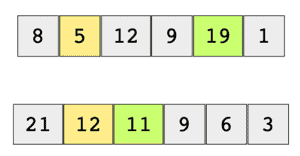# Find Maximum Single Sell Profit

## Problem Statement

Given a list of daily stock prices (integers for simplicity), return the buy and sell prices for making the maximum profit.

We need to maximize the single buy/sell profit. If we can’t make any profit, we’ll try to minimize the loss. For the below examples, buy (orange) and sell (green) prices for making a maximum profit are highlighted.## Try it yourself

tuple<int, int> find_buy_sell_stock_prices(int* array, int size) {
//TODO: Write - Your - Code
tuple<int, int> result(std::make_pair(-1, -1));
return result; // t is a tuple with (high, low) price values
}

## Solution

tuple<int, int> find_buy_sell_stock_prices(int* array, int size) {
if(array == NULL || size < 2) {
tuple<int, int> t(std::make_pair(NULL, NULL));
return t;
}

int global_sell = array;
int global_profit = global_sell - current_buy;

int current_profit = -2147483648; //INT_MIN

for(int i = 1; i < size; i++) {

if(current_profit > global_profit) {
global_profit = current_profit;
global_sell = array[i];
}

}
}

tuple<int, int> result(std::make_pair(
global_sell                  //sell price
));

return result;
}
int main() {
int array[] = {1, 2, 3, 4, 3, 2, 1, 2, 5};
tuple<int, int> result;
cout << "Buy Price: "<< get<0>(result) << ", Sell Price: " << get<1>(result) << endl;

int array2[] = {8, 6, 5, 4, 3, 2, 1};
cout << "Buy Price: " << get<0>(result) << ", Sell Price: " << get<1>(result) << endl;
}

## Solution Explanation

### Runtime complexity

The runtime complexity of this solution is linear, O(n).

### Memory complexity

The memory complexity of this algorithm is constant, O(1).

### Solution Breakdown

The values in the array represent the cost of a stock each day. As we can buy and sell the stock only once, we need to find the best buy and sell prices for which our profit is maximized (or loss is minimized) over a given span of time.

A naive solution, with runtime complexity of $O(n^{2})$, is to find the maximum gain between each element and its succeeding elements.

There is a tricky linear solution to this problem that requires maintaining current_buy_price (which is the smallest number seen so far), current_profit, and global_profit as we iterate through the entire array of stock prices. At each iteration, we will compare the current_profit with the global_profit and update the global_profit accordingly.

The basic algorithm is as follows:

current profit = INT_MIN
global sell = stock_prices
global profit = global sell - current buy

for i = 1 to stock_prices.length:
current profit = stock_prices[i] - current buy
if current profit is greater than global profit
then update global profit to current profit and update global sell to stock_prices[i]
if stock_prices[i] is less than current buy
then update current buy to stock_prices[i]

return global profit and global sell


Learn in-demand tech skills in half the time